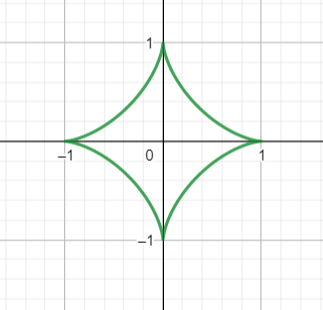# Let C be the curve parameterized by p(t)=[(\cos(t))^3,(\sin(t))^3], where 0 \leq t \leq 2...

## Question:

Let {eq}C {/eq} be the curve parameterized by {eq}\displaystyle p(t) = [(\cos(t))^3, (\sin(t))^3], {/eq} where {eq}\displaystyle 0 \leq t \leq 2 \pi. {/eq}

a) Use MATLAB to plot the curve.

b) Calculate by hand the speed of the curve.

## Space Curves:

We typically use a vector parameterization to describe a curve in real physical space. Plotting curves these days is much easier than it used to be since there is a variety of graphing software out there that will pretty much do it for us. We will plot the curve, then use differentiation to find its velocity (recall that the derivative of the position is the velocity).

Part A

We use our favorite graphing software to plot the curve.{eq}\begin{align*} \vec p (t) &= \left< \cos^3 t, \sin^3 t \right> \end{align*} {/eq}

Part B

The velocity is the derivative of the position and the magnitude of the velocity is the speed. We find

{eq}\begin{align*} \vec v (t) &= \frac{d}{dt} \left< \cos^3 t, \sin^3 t \right> \\ &= \left< -3\cos^2 t \sin t, 3 \cos t \sin^2 t \right> \\ &= 3 \left< -\cos^2 t \sin t, \cos t \sin^2 t \right> \end{align*} {/eq}

And so the speed is

{eq}\begin{align*} | \vec v (t) | &= 3 \sqrt{(-\cos^2 t \sin t)^2+(\cos t \sin^2 t )^2} \\ &= 3 \sqrt{\cos^4 t \sin^2 t + \cos^2 t \sin^4 t} \\ &= 3 \sqrt{ \cos^2 t \sin^2 t (\cos^2 t + \sin^2 t)} \\ &= 3 \sqrt{ \cos^2 t \sin^2 t} \\ &= 3\ | \cos t \sin t| \end{align*} {/eq}